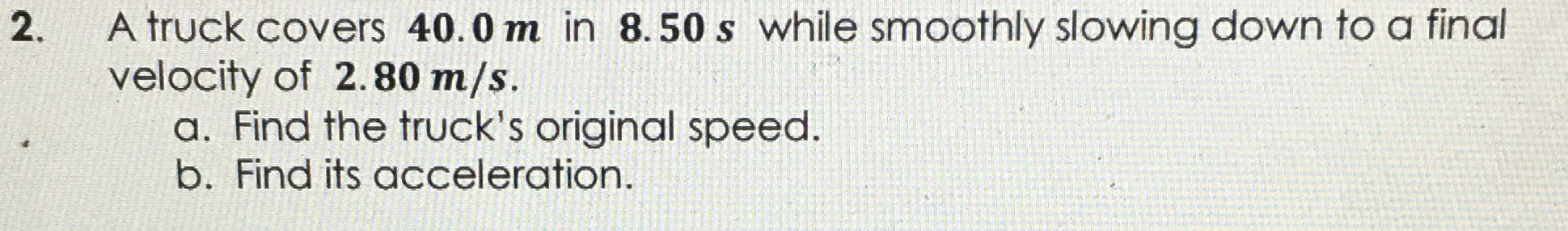### ¿Todavía tienes preguntas de matemáticas?

Pregunte a nuestros tutores expertos
Algebra
Pregunta2. A truck covers $$40.0 m$$ in $$8.50 s$$ while smoothly slowing down to a final velocity of $$2.80 m / s$$ . a. Find the truck's original speed. b. Find its acceleration.

a. original speed = 6.6 ms$$^{- 1}$$
b. acceleration = - 0.4 ms$$^{- 2}$$